# SAT Math Multiple Choice Question 980: Answer and Explanation

### Test Information

Question: 980

16.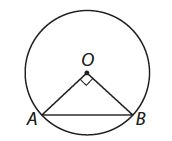In the figure above, circle O has a circumference of 12π. If AB = 8, what is BC

?
• A.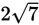• B.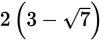• C.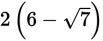• D.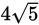Explanation:

B

Difficulty: Hard

Category: Additional Topics in Math / Geometry

Strategic Advice: Identify pieces of the figure that play roles in multiple shapes present (for example, a radius of the circle that is also a leg of a triangle), and transfer information as you work toward the answer.

Getting to the Answer: The circumference of circle O is 12π, so its radius must be 6(C = 2πr). You now know the lengths of two sides of ΔAOB (the given hypotenuse and the leg represented by the radius), and because it's a right triangle, you can use the Pythagorean theorem to find the length of the missing side (OB):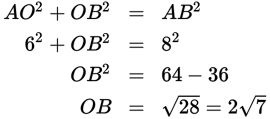Don't stop yet; the question asks for BC, not OB. BC is equal to 6 - 2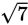, which is (because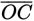is also a radius). Factor out a 2 to get, which matches (B).